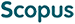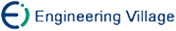王海霞 韩承德. 整数乘法电路的形式化验证方法研究[J]. 计算机研究与发展, 2005, 42(3).
 引用本文: 王海霞 韩承德. 整数乘法电路的形式化验证方法研究[J]. 计算机研究与发展, 2005, 42(3).Wang Haixia and Han Chengde. Formal Method Research on Integer Multiplier Verification[J]. Journal of Computer Research and Development, 2005, 42(3).
 Citation: Wang Haixia and Han Chengde. Formal Method Research on Integer Multiplier Verification[J]. Journal of Computer Research and Development, 2005, 42(3).## Formal Method Research on Integer Multiplier Verification

Abstract: Model checking based on decision diagram causes memory explosion in integer multiplier verification. An efficient solution to this problem is backward substitution method. As the kernel of backward substitution method, the performance of function substitution algorithm is crucial to the efficiency of verification process using backward substitution method. If the variable to be substituted is ensured to be the top variable of the function to be substituted, the function substitution algorithm can be simplified. By setting variable order and variable substitution order, an improved backward substitution method is presented, where in each substitution, the variable substituted is the top variable of the decision diagram of function to be substituted. As experiment shows, under 1GB memory, the improved method enhances the scale of Add-Step multiplier which can be verified from 84×84 bits to 256×256 bits，and Diagonal multiplier from 84×84 bits to 206×206 bits./下载:  全尺寸图片 幻灯片
• 分享
• 用微信扫码二维码

分享至好友和朋友圈